﻿﻿﻿ Chapter Walkthrough Chapter Walkthrough | Scholastic International

### Chapter Walkthrough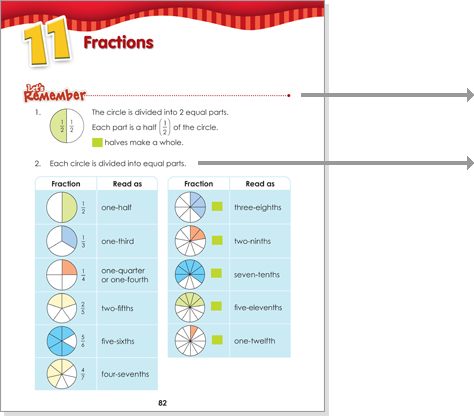•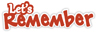At the beginning of a chapter, Let's Remember assesses students' recall and mastery of precursor knowledge.

• Tasks in Let's Remember provide feedback on readiness to learn new knowledge or need for remediation.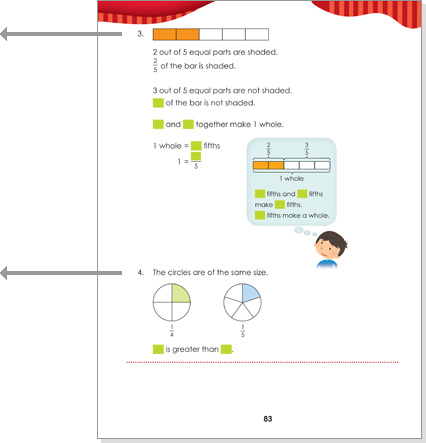• Objective and chapter reference of each task are provided in Teacher's Guide if there is a need for remediation.

• Tasks in Let's Remember are systematically varied to ensure full review.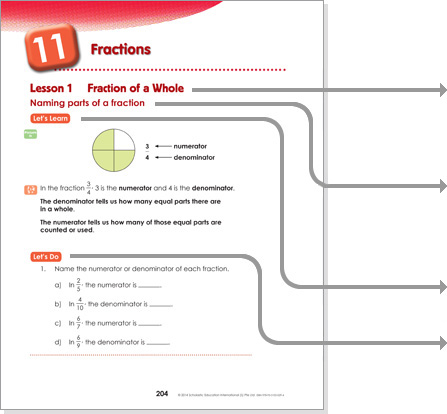• Each chapter is taught over several lessons, with each lesson focusing on a topic.
• Each lesson teaches one or more concepts. Each concept is taught using a two-part structure of Let’s Learn and Let’s Do.
• Let’s Learn introduces the concept using the Concrete-Pictorial-Abstract approach.
• Let’s Do provides guided practice and formative assessment.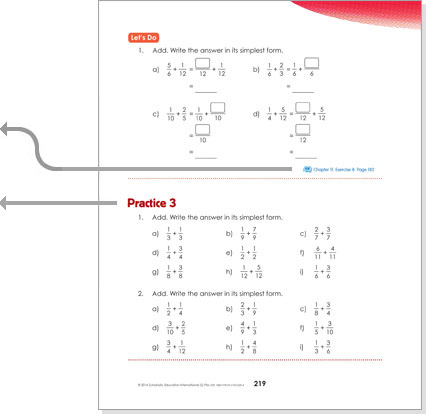• Practice Book exercises reinforce learning and provide independent practice.
• At the end of each lesson, Practice consolidates learning and provides summative assessment.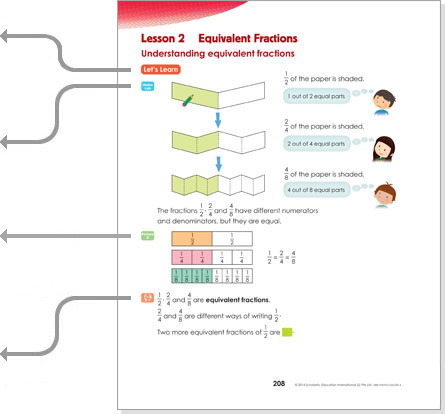• Each concept in Let's Learn is taught using the three-stage Concrete-Pictorial-Abstract approach.
• Concrete: Physical materials are used in hands-on activities to develop conceptual understanding.
• Pictorial: Visuals are used to represent physical materials previously used to connect mathematical ideas.
• Abstract: Number and mathematical symbols are used as a final presentation of concepts and skills.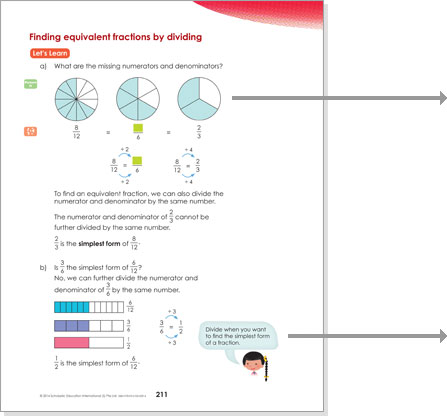• Consistent lesson design and icons make lesson delivery intuitive for teachers.

• Speech bubble provides prompt for teacher to guide students' thought process.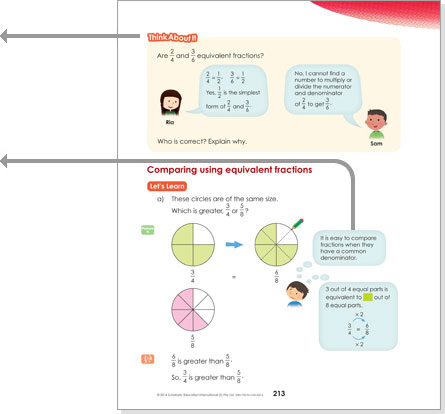• Think About It develops metacognitive skills through mathematical reasoning and justification.

• Thought bubble models the thinking process to train students to monitor their own thinking.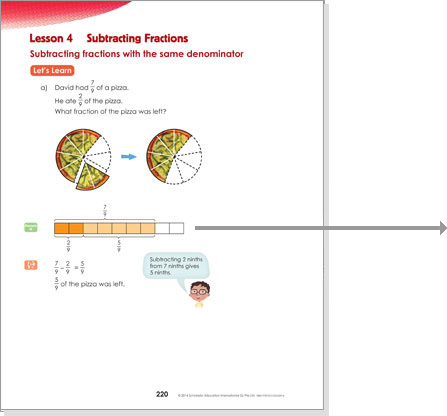• The Bar Model Method allows students to solve complex word problems using visual representation.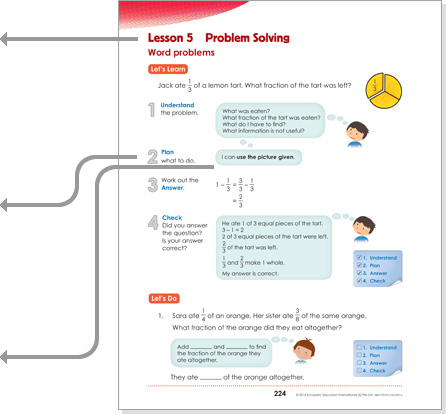• At the end of each chapter,
a problem solving lesson consolidates learning.

• Explicit teaching of the four-step UPAC process builds good problem solving habits.

• Explicit teaching of nine heuristics equips students to tackle different types of word problems.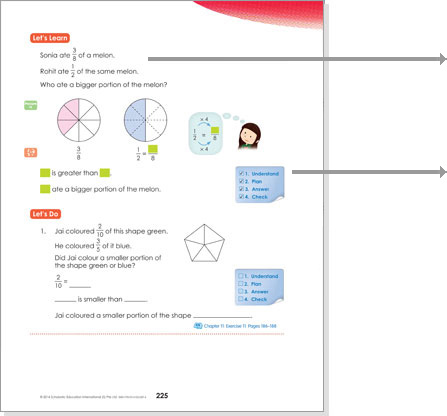• Word problems enable students to apply the concepts learnt and make mathematics relevant to daily life.

• A checklist accompanies every word problem to reinforce the four-step process.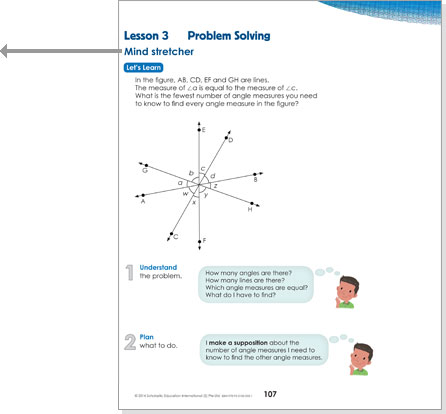• Mind Stretcher is a non-routine task to develop higher order thinking skills.